Next: Isotone Relations Up: Reduction Theorems Previous: Cores

## Fixed Point Theorems/Reflection Conditions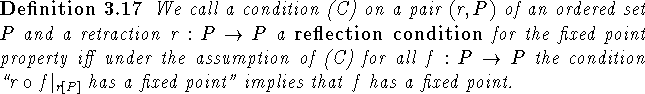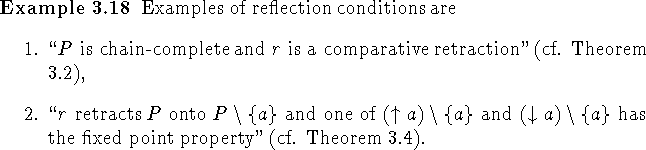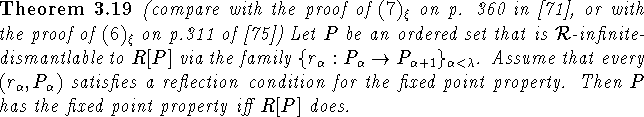Proof: First note that since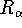is a retraction the condition ``P has the fixed point property" implies that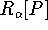has the fixed point property for all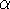.
For the other direction let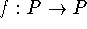be order-preserving. Then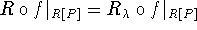has a fixed point p. We will prove inductively for allthat the condition ``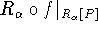has a fixed point p" implies that f has a fixed point. For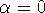this is trivial. Now let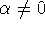and assume the assertion holds for all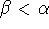. First supposeis a successor ordinal. If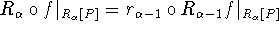has a fixed point then (since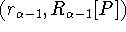satisfies a reflection condition)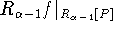has a fixed point. By induction hypothesis the latter implies that f has a fixed point.
Finally ifis a limit ordinal suppose thathas a fixed point p. Then there is a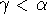such that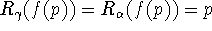. Thus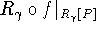has p as a fixed point and by induction hypothesis f has a fixed point. \

Theorem 3.19 obviously leads to an algorithm for a sufficient condition for the fixed point property: Dismantle P via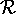-retractions as far as possible and verify a reflection condition at every step. Any set for which this is possible with a core that has the fixed point property has the fixed point property itself. The difficulty in finding reflection conditions however is considerable as can be seen in . Infinite-dismantlability also gives an algorithmic insight into a classical result about the fixed point property for ordered sets of height 1 (recall that the height of an ordered set P is the number of elements in the largest chain in P minus 1):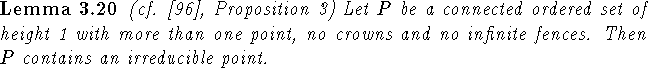Proof: Assume P does not contain an irreducible point. Let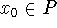be arbitrary and minimal. Let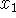be an upper cover of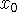. Assume that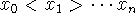have already been chosen and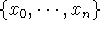is a fence. We will assume without loss of generality that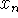is minimal. Thenhas an upper cover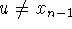. Choose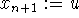. Then since P does not contain any crowns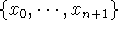is a fence. In this fashion we can construct an infinite fence in P, contradiction. \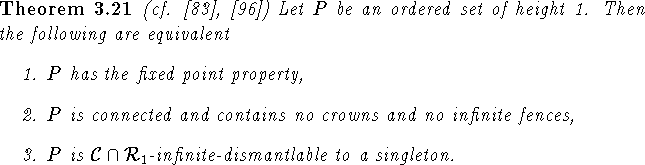Proof: ``1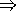2": Necessity of connectedness is trivial. If P contains a crown P cannot have the fixed point property as is proved in , p. 312 ff. (the argument given never uses that P is finite). Thus P contains no crowns. Were P to contain an infinite fence F and no crowns, then F is an isometric spanning fence and hence by Theorem 2 in  it is a retract (this is also easy to see directly). This would again imply that P does not have the fixed point property. Hence 2 must hold.
``23": Let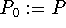. Suppose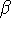is an ordinal number and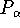as in Definition 3.7 have already been defined for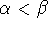. Ifhas a predecessor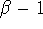such that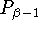is not a singleton, then there is a point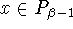that is irreducible. Let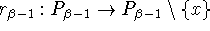be the retraction that removes x and let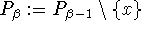. Ifis a limit ordinal notice that since P does not contain any infinite fences for each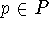the sequence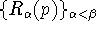as in Definition 3.7 must be constant for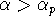for some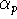. We define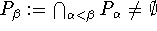. Ifis a singleton we stop. The sequence thus generated is an infinite dismantling of P.
``31" follows from Theorem 3.19. \

Theorem 3 in , which is used to prove Theorem 4 in  (which is Theorem 3.21 here) can now be obtained via one of the standard translation processes between ordered sets and graphs (similar to what is done in ).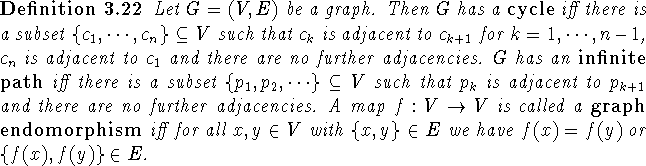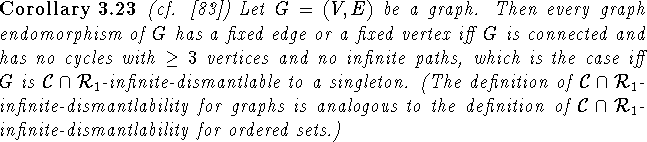Proof: (Compare with the idea of the proof of Theorem 2.14.) Let G=(V,E) be a graph. We define an ordered set of height 1 with underlying set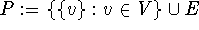and with the order being containment of sets. Let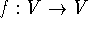be a graph endomorphism of G. Then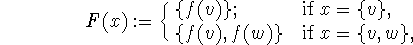is an order-preserving map of P. Moreover if F has a fixed point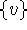that is minimal in P, then f fixes v. If F fixes no singleton, but has a fixed point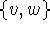that is maximal in P, then f fixes. Thus if P has the fixed point property, then every endomorphism of G fixes an edge or a vertex.
Let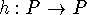be an order-preserving map of P that maps the minimal elements to minimal elements. If for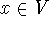we let H(x) be the unique element of the singleton set h(x), then H is a graph endomorphism of G. Moreover if H has a fixed vertex, then clearly h has a fixed point, and if H has a fixed edge, then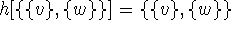and hence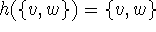. Thus if every endomorphism of G has a fixed vertex or a fixed edge, then every order-preserving map of P that maps minimal elements to minimal elements has a fixed point and consequently P has the fixed point property.
Hence P has the fixed point property iff every endomorphism of G has a fixed edge or a fixed vertex. However by Theorem 3.21 P has the fixed point property iff P is connected and has no crowns and no infinite fences. This is the case exactly when G is connected and has no cycles with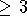elements and no infinite paths.
Finally note that G is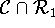-infinite-dismantlable to a singleton iff P is. \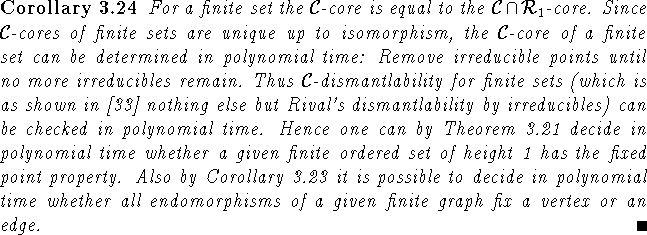Next: Isotone Relations Up: Reduction Theorems Previous: Cores

Bernd.S.W.Schroder